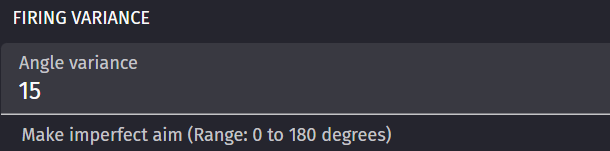hi, I was testing an example where the “fire bullet” extension is present and I focused on how I could increase this “angle variance” option in my project.this is an example of shooting the bullet present in my project:

How can I increase this function without using the fire bullet extension?

If you create your own variance scene variable, in this example say we make variance = 15

When you spawn the bullet you could make the angle of the bullet = `RandomInRange(arma1.Angle() - (variance / 2), arma1.Angle + (variance / 2))`

This will have the bullet stray either 7.5 degrees to the left or right (15 degrees variance total).

Then you can use the bullets angle to determine the direction it will fire.

Hope this helps.

1 Like

If I may, I believe you are meant to apply the formula to the part that says to change the angle of the bullet, like so:

Change the angle of bullet1: set to RandomInRange((arma1.Angle() - (variance / 2)), (arma1.Angle() + (variance / 2)))

For the part with the force angle you just put there bullet1.Angle()

edit: Oh and I added in some parentheses, I’m not sure if my syntax is correct. Also now that I think about it, putting the formula in the force angle part might also work except that the bullets might be facing a different direction from where they’re going. You could probably figure it out.

Reference sample:

1 Like

ok thanks guys it works now

1 Like